### Is Deep Learning Secure for Robots?

Han Wu, Dr. Johan Wahlström and Dr. Sareh Rowlands, University of Exeter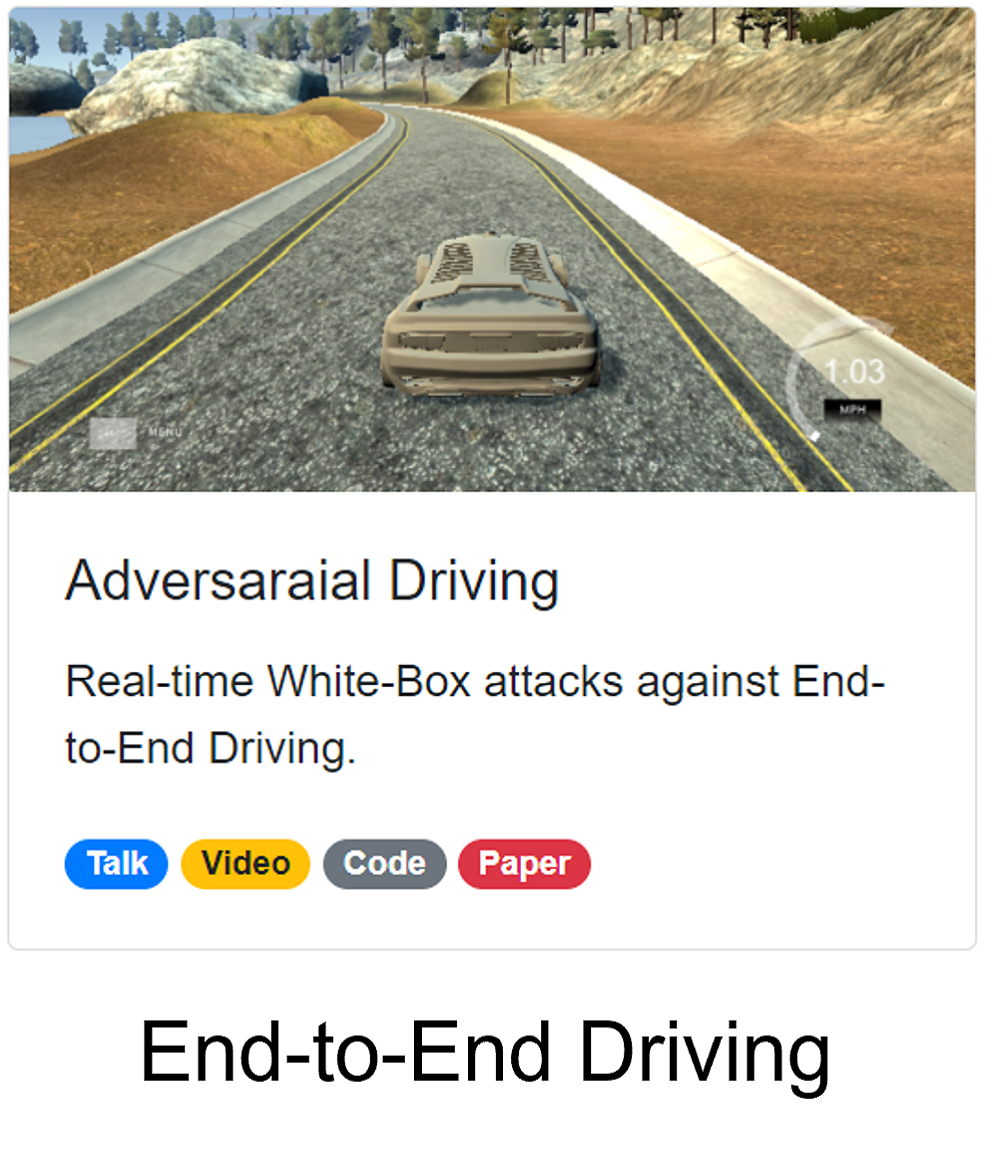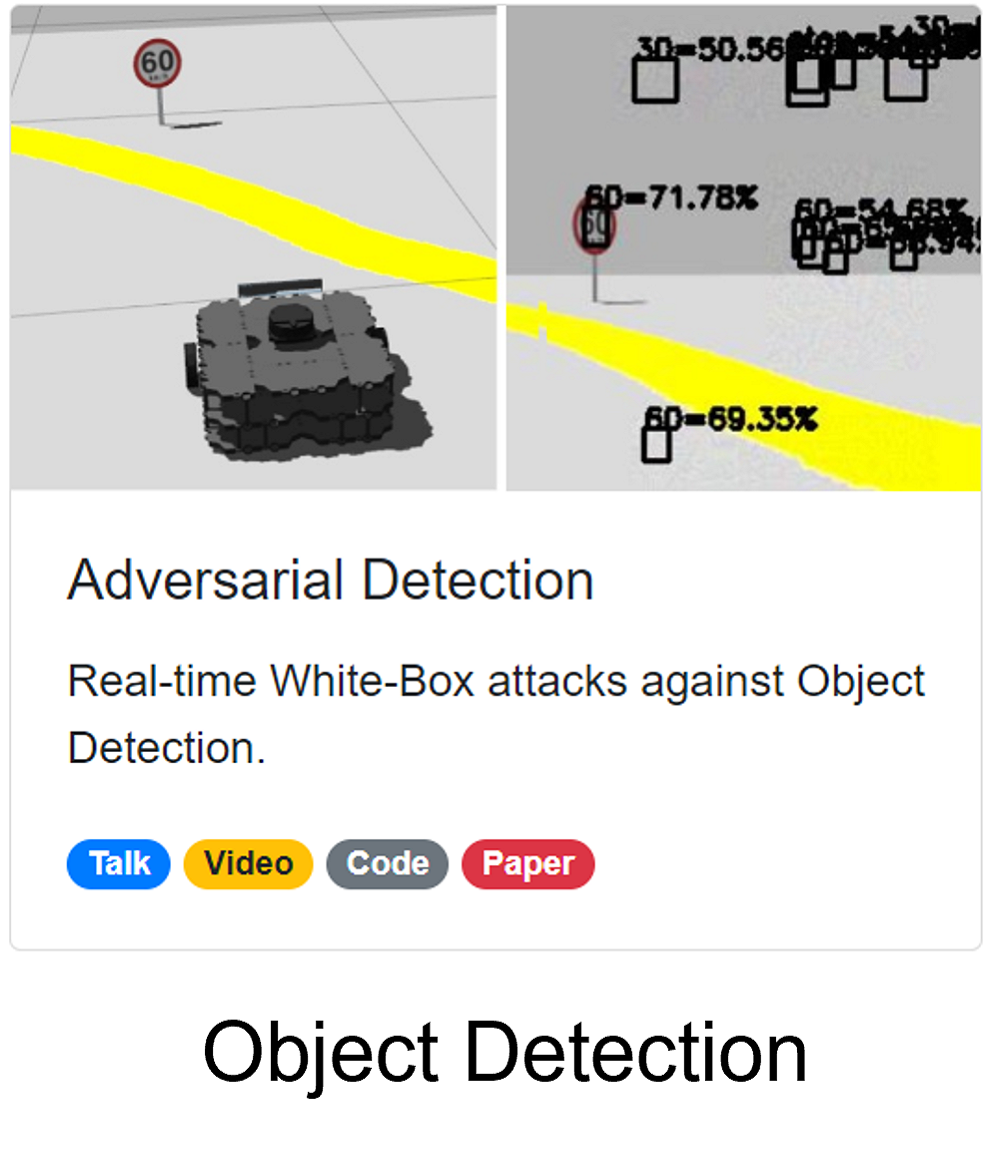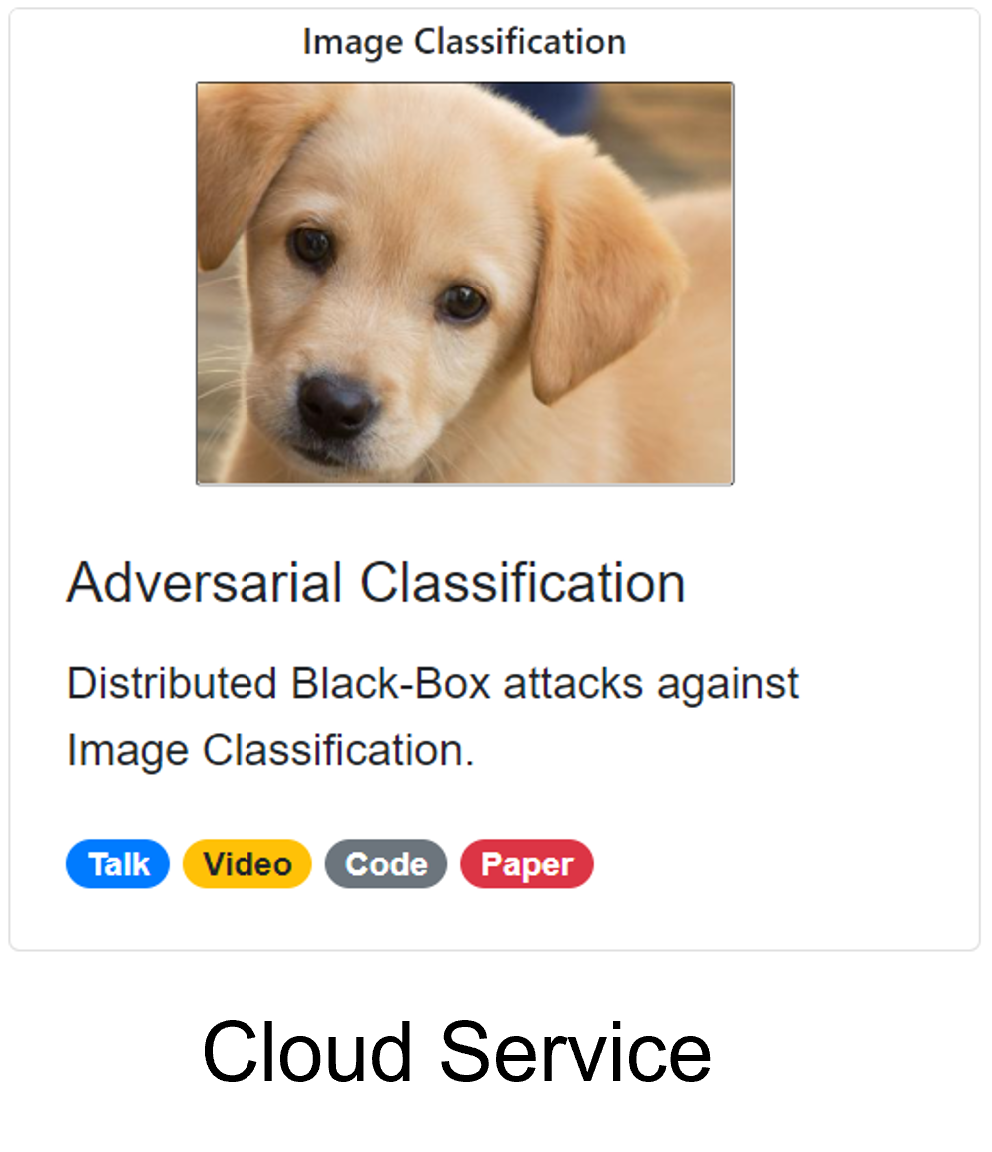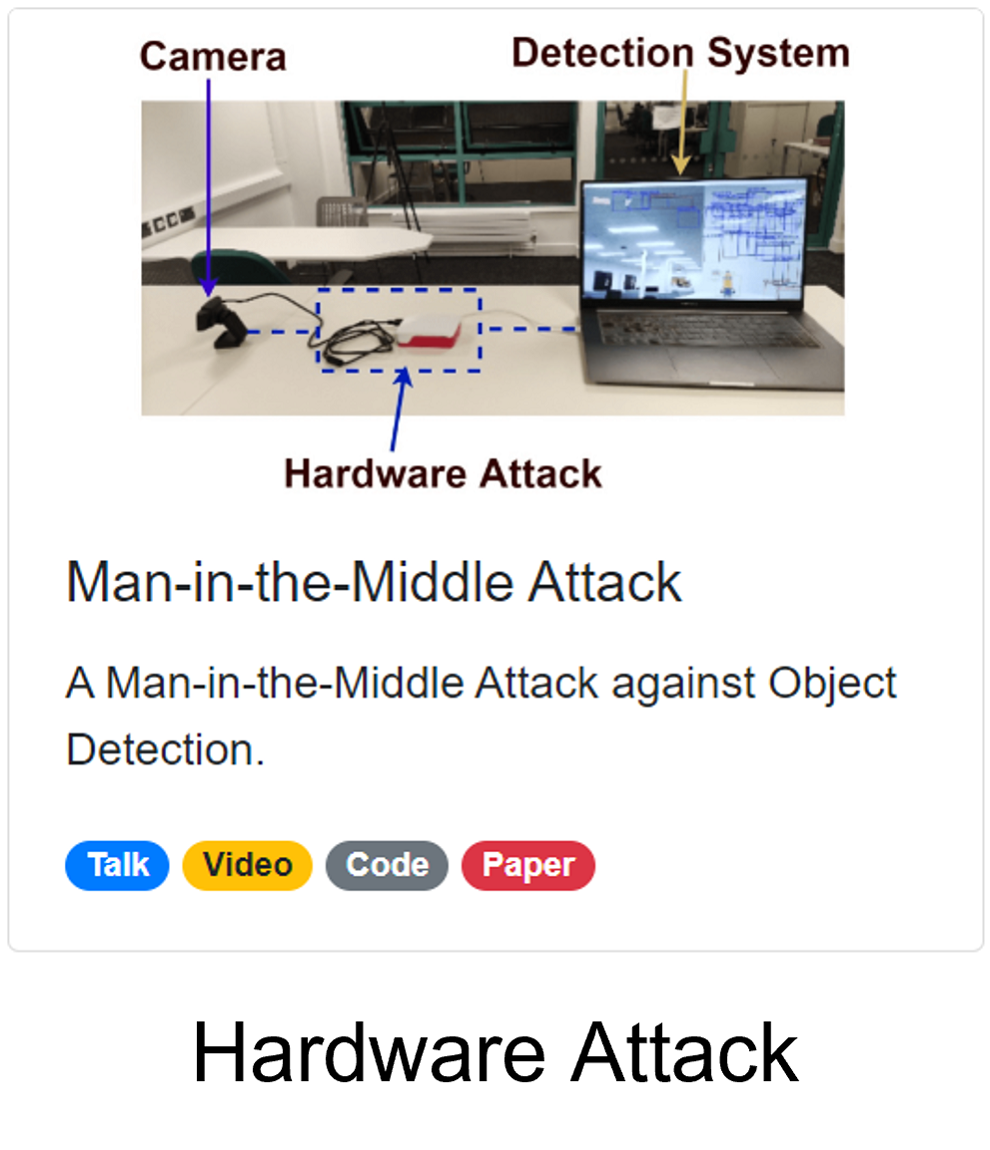Attacking End-to-End Driving Models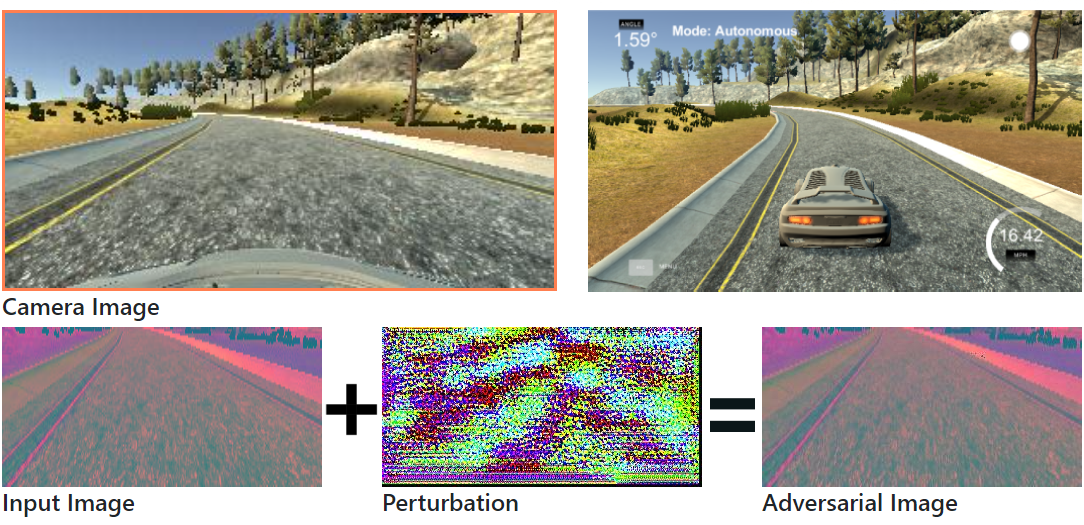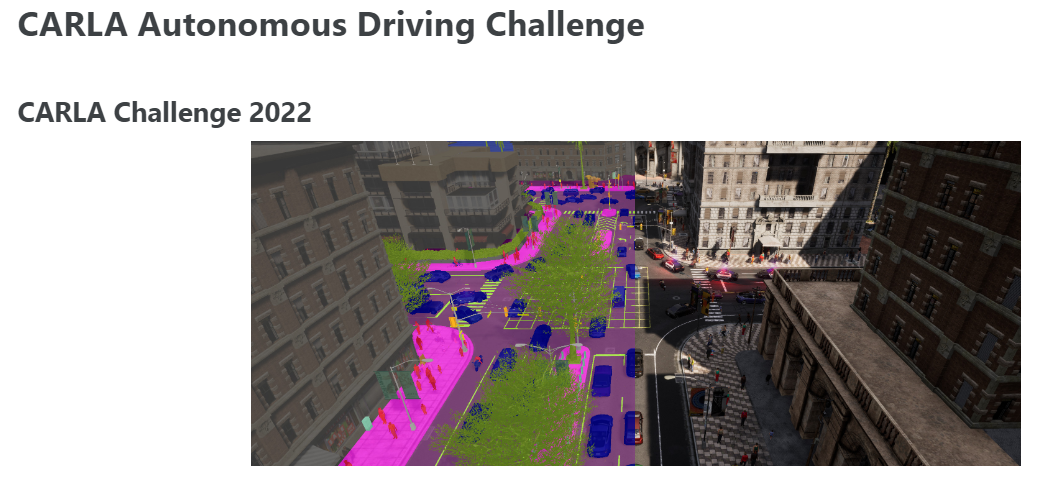• Almost all the top 10 teams on the leaderboard use end-to-end driving models.
• End-to-End driving models lead to smaller systems and better performance.

##### Adversarial Attacks against End-to-End Driving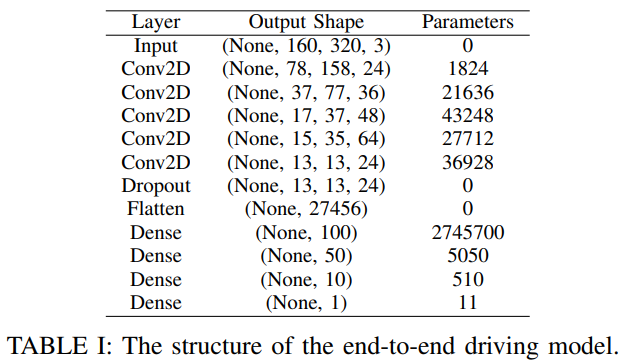The NVIDIA End-to-End Driving ModelAdversarial attacks against image classification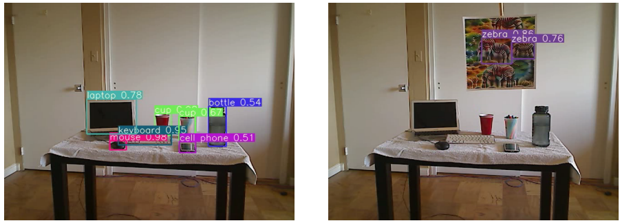Adversarial attacks against object detection

 J. Z. Kolter and A. Madry, Adversarial Robustness - Theory and Practice, NeurIPS 2018 tutorial.

##### Problem Definition
• Given an input image 𝑥 and the end-to-end driving model $y = f(\theta, x)$.
• Our objective is to generate an adversarial image $x^{'} = x + \eta$ such that:
• $$y^{'}=f(𝜃,x^{'}) \neq y$$
• To ensure that the perturbation is unperceivable to human eyes:
• $$\Vert x^{'}-x \Vert_2 = \Vert{\ \eta\ }\Vert_2 \leq \xi, \text{where } \xi=0.03$$
• For offline attacks, we can use pre-recorded human drivers' steering angles as the ground truth $y^*$.
• $$\eta = \epsilon\ sign(\nabla_x J(y,\ y^{*} ))$$
• For a real-time online attack, we do not have access to the ground truth $y^*$.
• $$\eta = \epsilon\ sign(\nabla_x J(y))$$

## Random Noises

### Image-Specific Attack

• Output: Steering Angle 𝑦∈[-1, 1]
• Decrease the output (left):
• $$J_{left}(y)= - y$$
• Increase the output (right):
• $$J_{left}(y)= y$$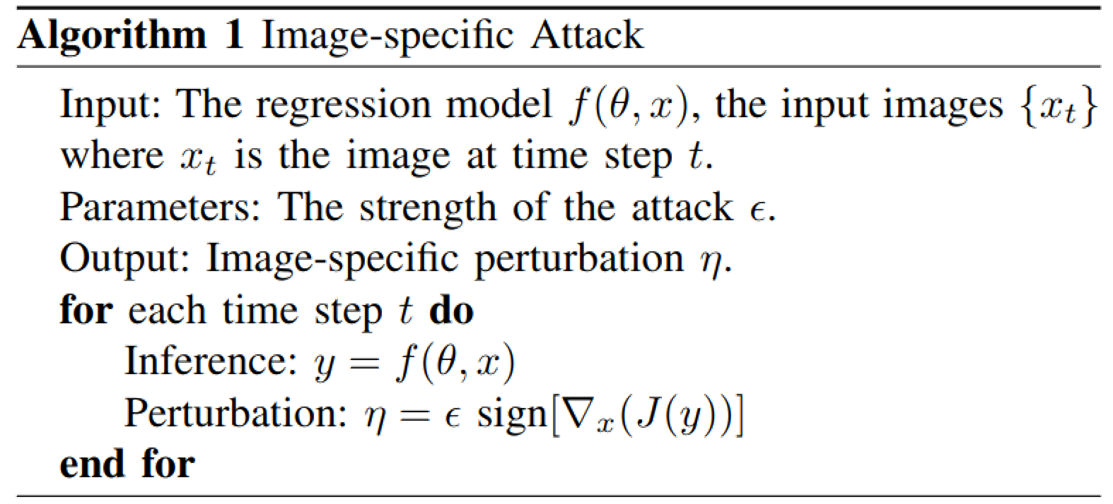### Image-Agnostic Attack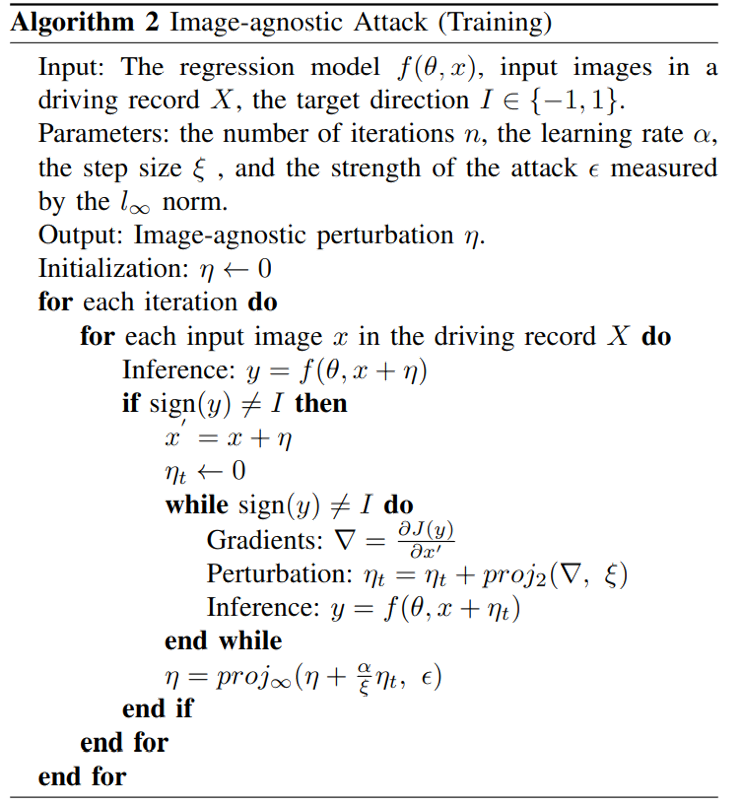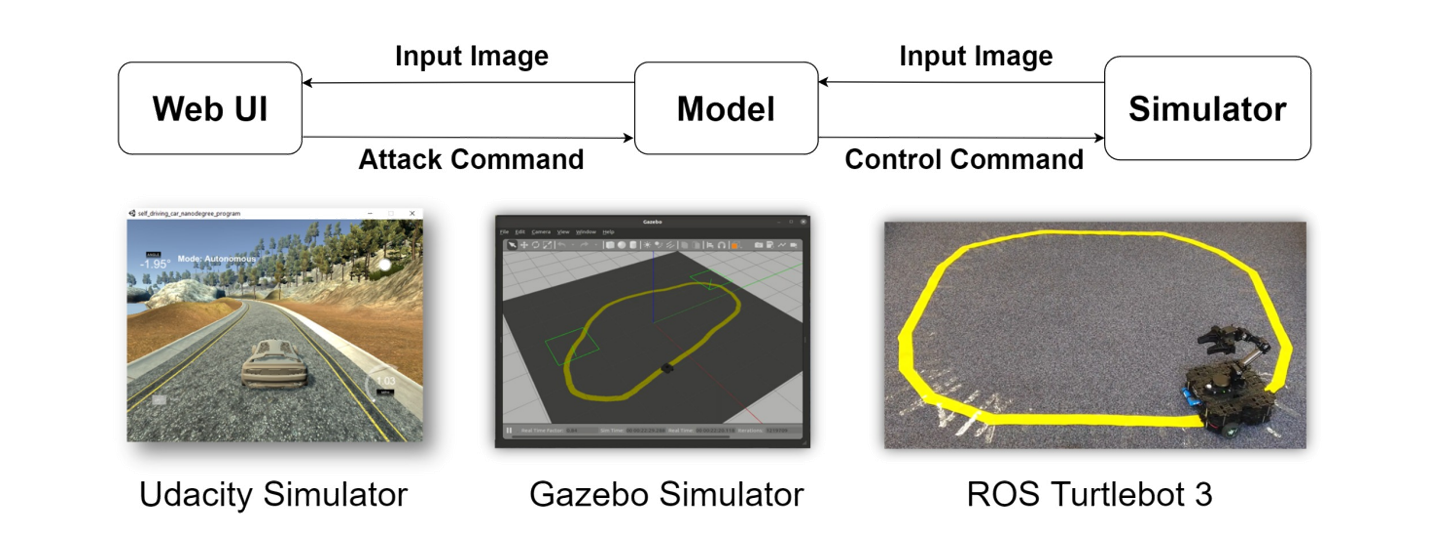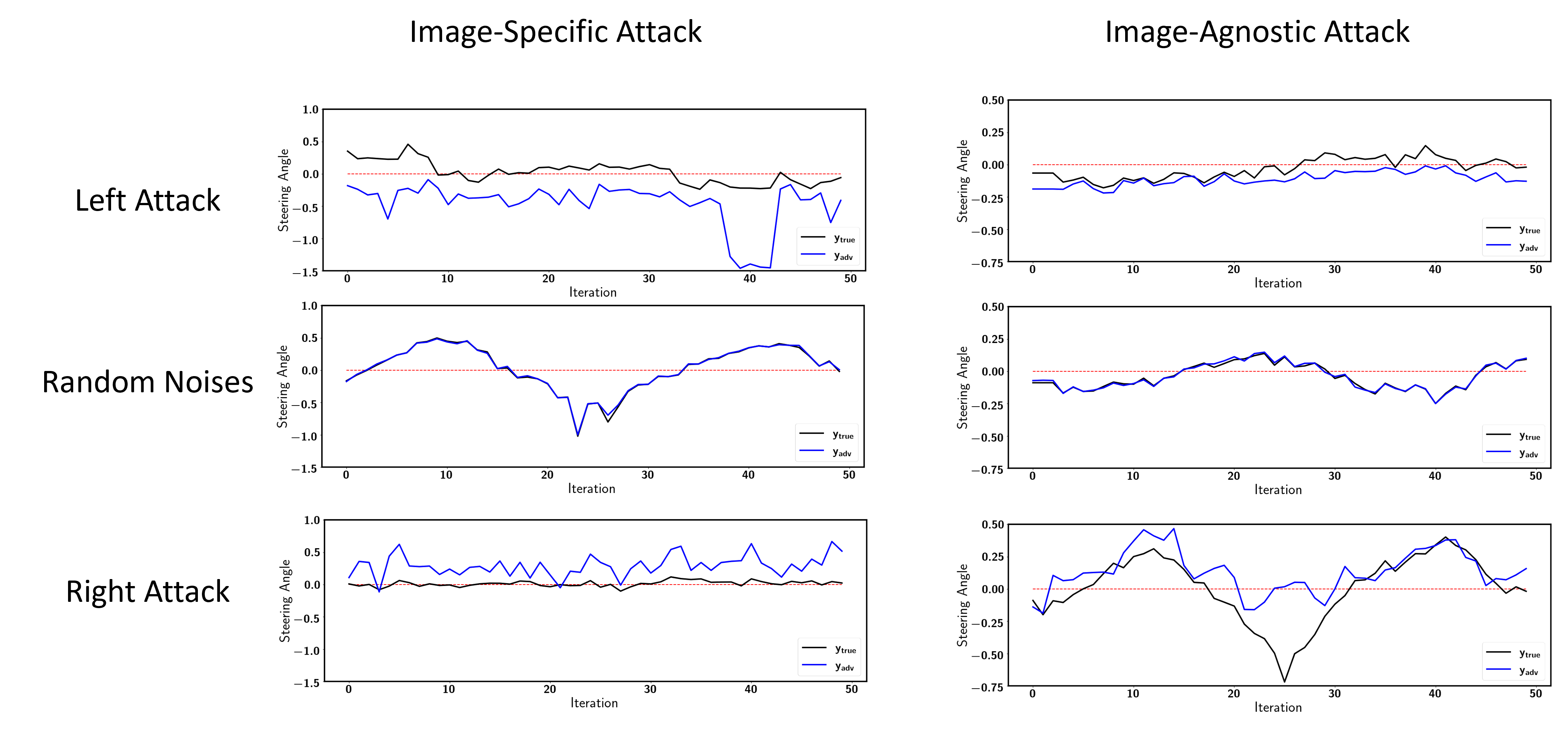Attacking Object Detection in Real Time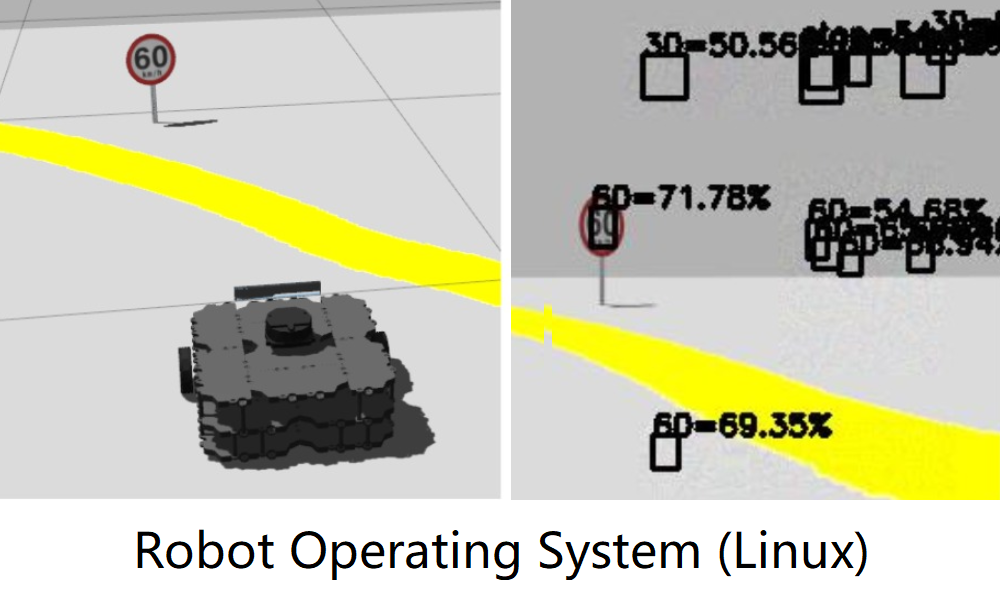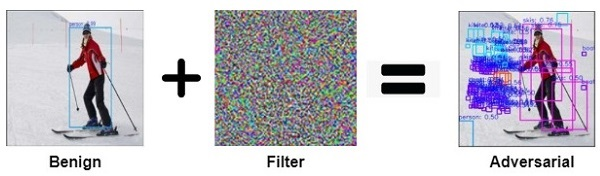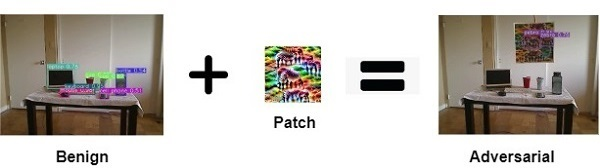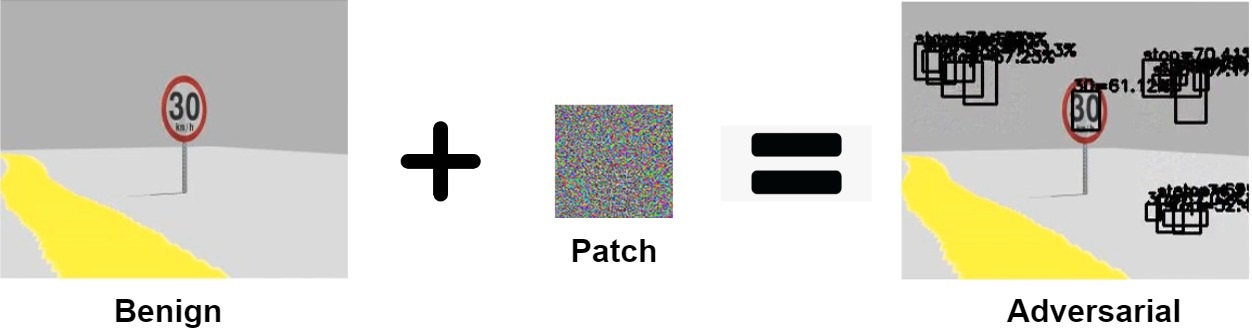How different attacks apply the perturbation $\delta$ using a binary mask $m \in \{0, 1\}^{wh}$

$x^{'}_{filter} = x + \delta$         $x^{'}_{overlay} = x + m \odot \delta$         $x^{'}_{patch} = (1-m) \odot x + m \odot \delta$

Given an input image $x$, the object detection model outputs $S \times S$ candidate bounding boxes $o \in \mathcal{O}$ at three different scales.

Each candidate box $o^i$ contains $(b_x^i, b_y^i, b_w^i, b_h^i, c^i, p_1^i, p_2^i, ..., p_K^i)$ for K classes, where $0 \leq i \leq |\mathcal{O}|$.

\begin{aligned} \text{One-Targeted}:\ \mathcal{L}_{adv}^{1}(\mathcal{O}) &= \max_{1 \leq i \leq |\mathcal{O}|}\ [\sigma(c^i) * \sigma(p^i_t)] \\ \text{Multi-Targeted}:\ \mathcal{L}_{adv}^{2}(\mathcal{O}) &= \sum^{|\mathcal{O}|}_{i = 1}\ [\sigma(c^i) * \sigma(p^i_t)] \\ \text{Multi-Untargeted}:\ \mathcal{L}_{adv}^{3}(\mathcal{O}) &= \sum^{|\mathcal{O}|}_{i = 1} \sum_{j=1}^{K}\ [\sigma(c^i) *\sigma(p^i_j)] \end{aligned}

where $|\mathcal{O}| = \sum_{1 \leq i \leq 3} S_i \times S_i \times B$, and $S_i$ represents the grid size of the $i_{th}$ output layer ($S \in \{13,26,52\}$, $B=3$).

Distributed Black-box Attacks against Image Classification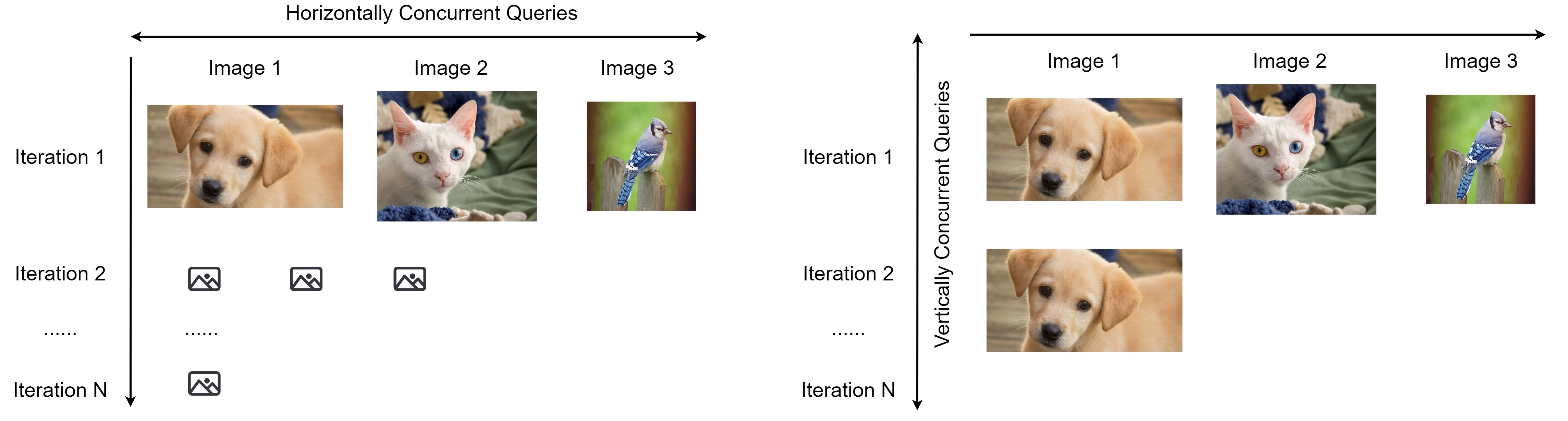### DeepAPI - The Cloud API we attack

We open-source our image classification cloud service for research on black-box attacks.

### DeepAPI Deployment

Using Docker

                        
$docker run -p 8080:8080 wuhanstudio/deepapi Serving on port 8080...   Using Pip  $ pip install deepapi

\$ python -m deepapi
Serving on port 8080...



### How to accelerate Black-Box attacks?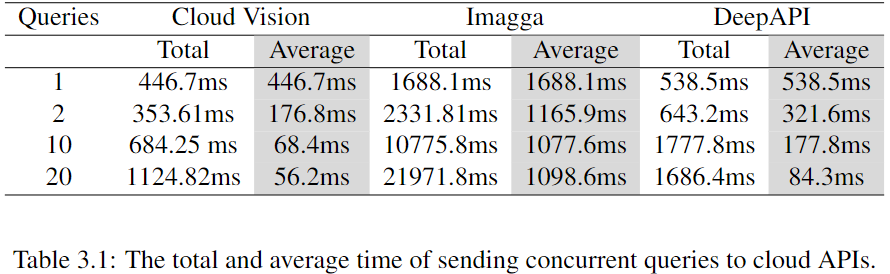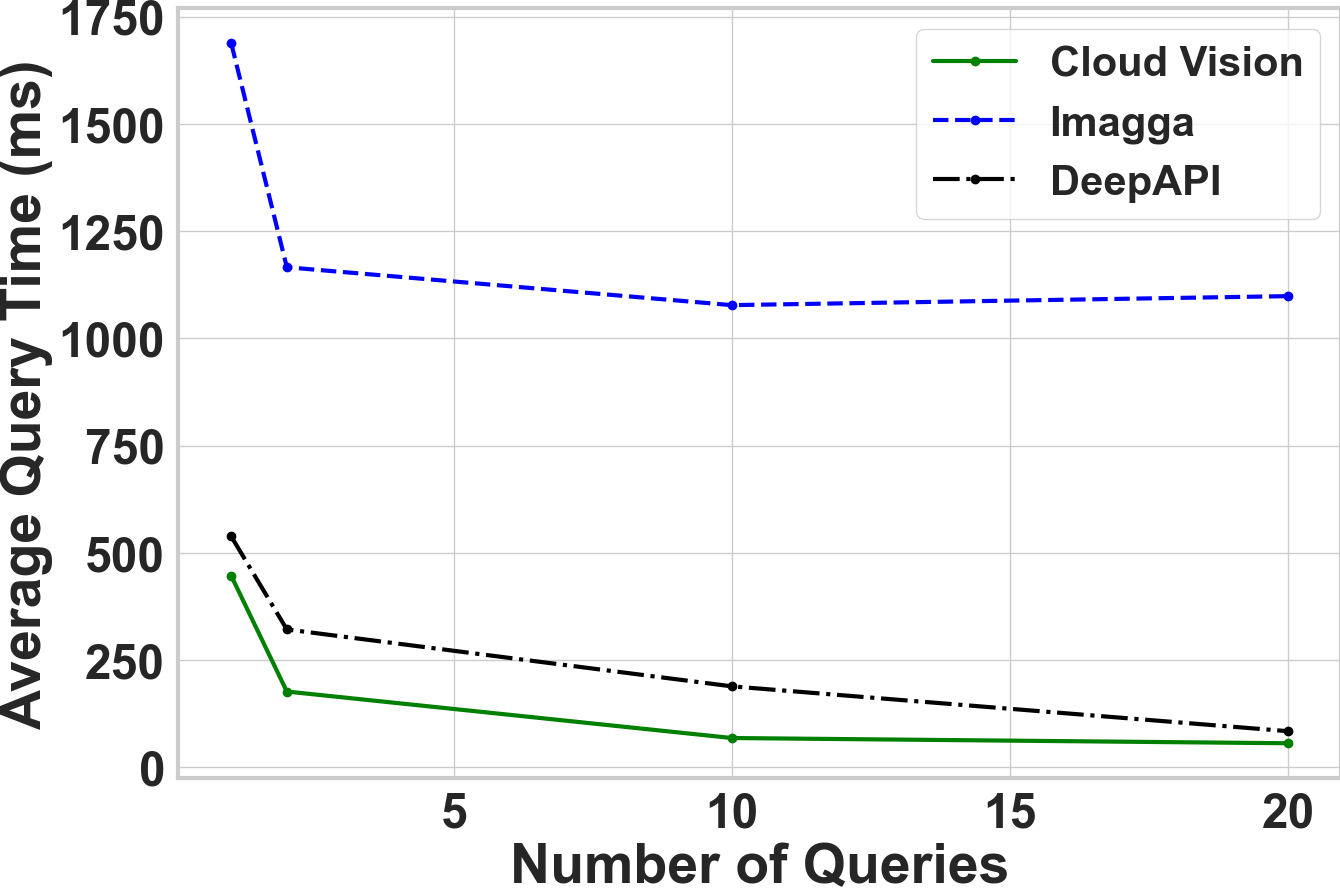Cloud APIs are deployed behind a load balancer that distributes the traffic across several servers.

### Local Models & Cloud APIs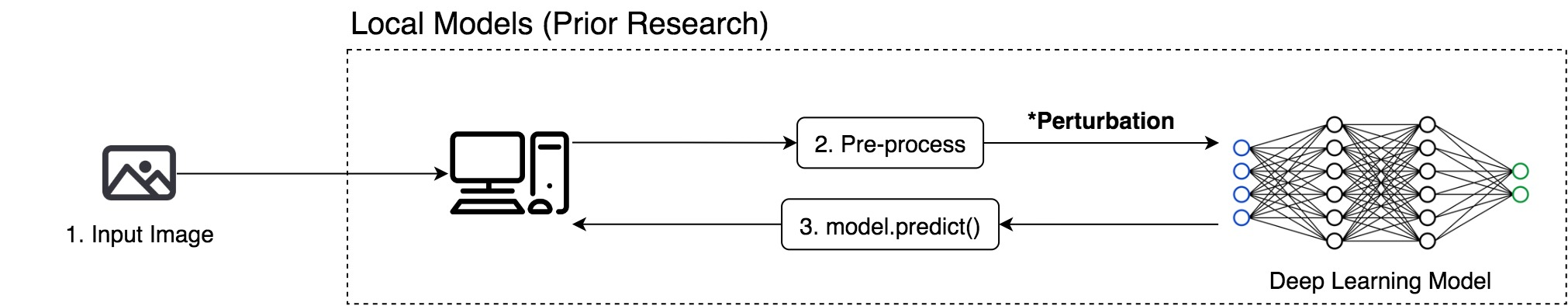Most prior research used local models to test black-box attacks.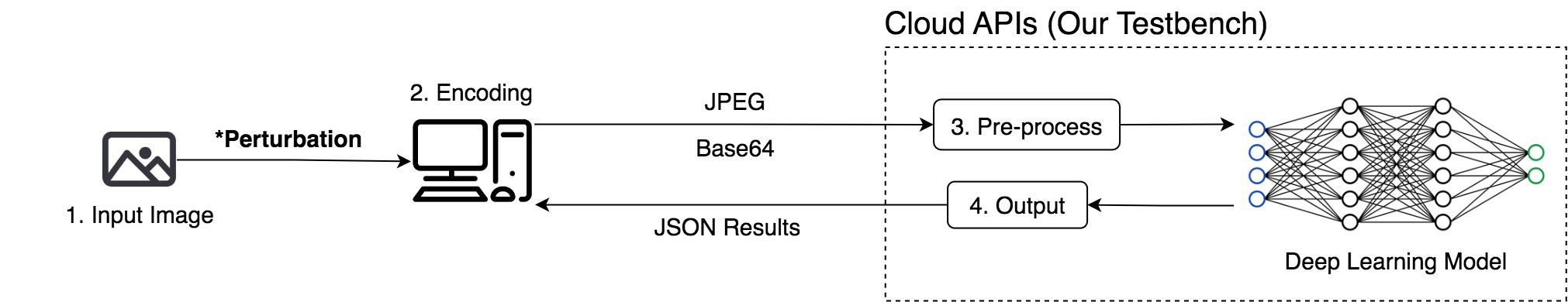We initiate the black-box attacks directly against cloud services.

### Attacking Cloud APIs is more challenging than attacking local models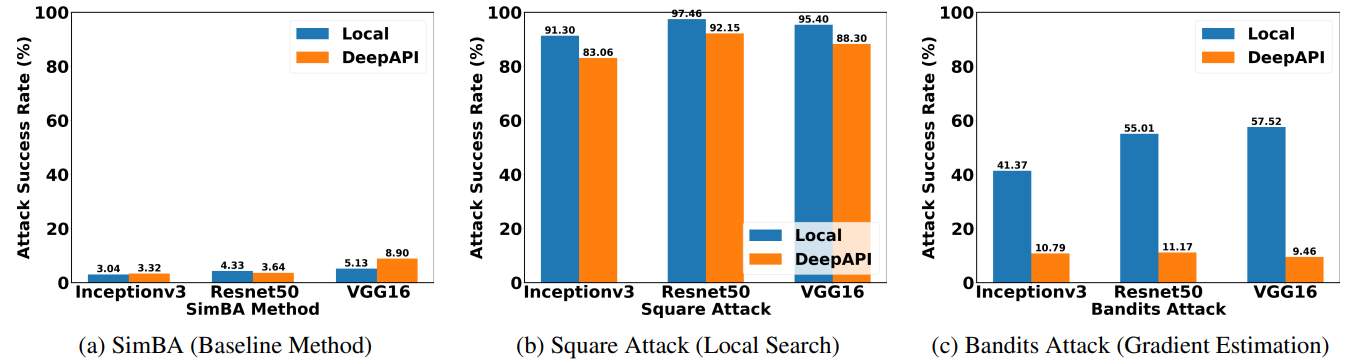Attacking cloud APIs achieve less success rate than attacking local models.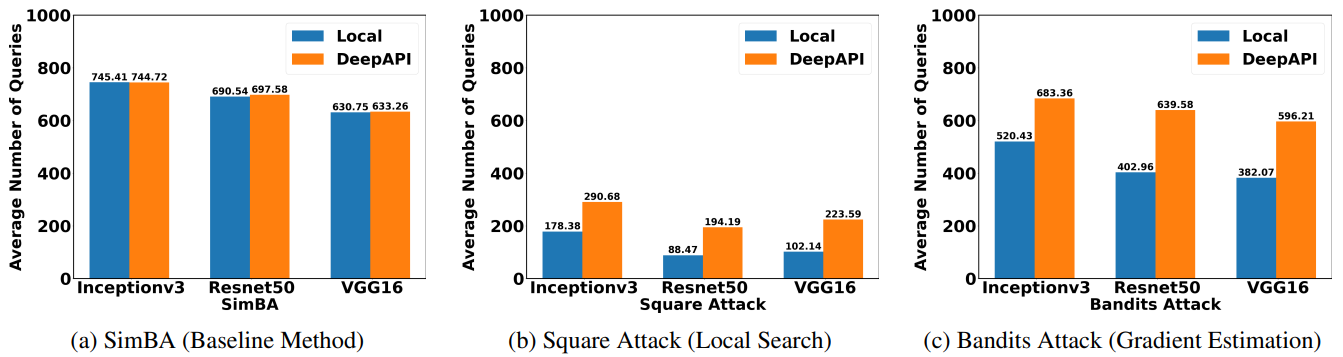Attacking cloud APIs requires more queries than attacking local models.

### Horizontal Distribution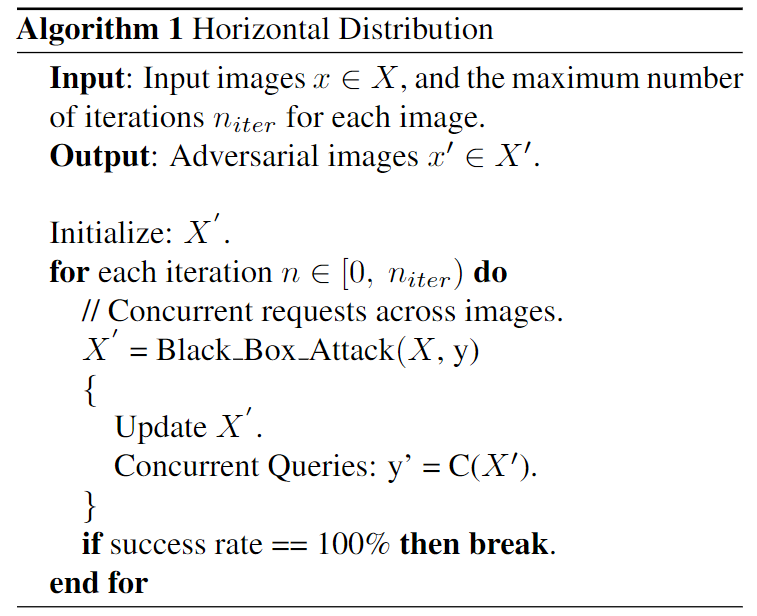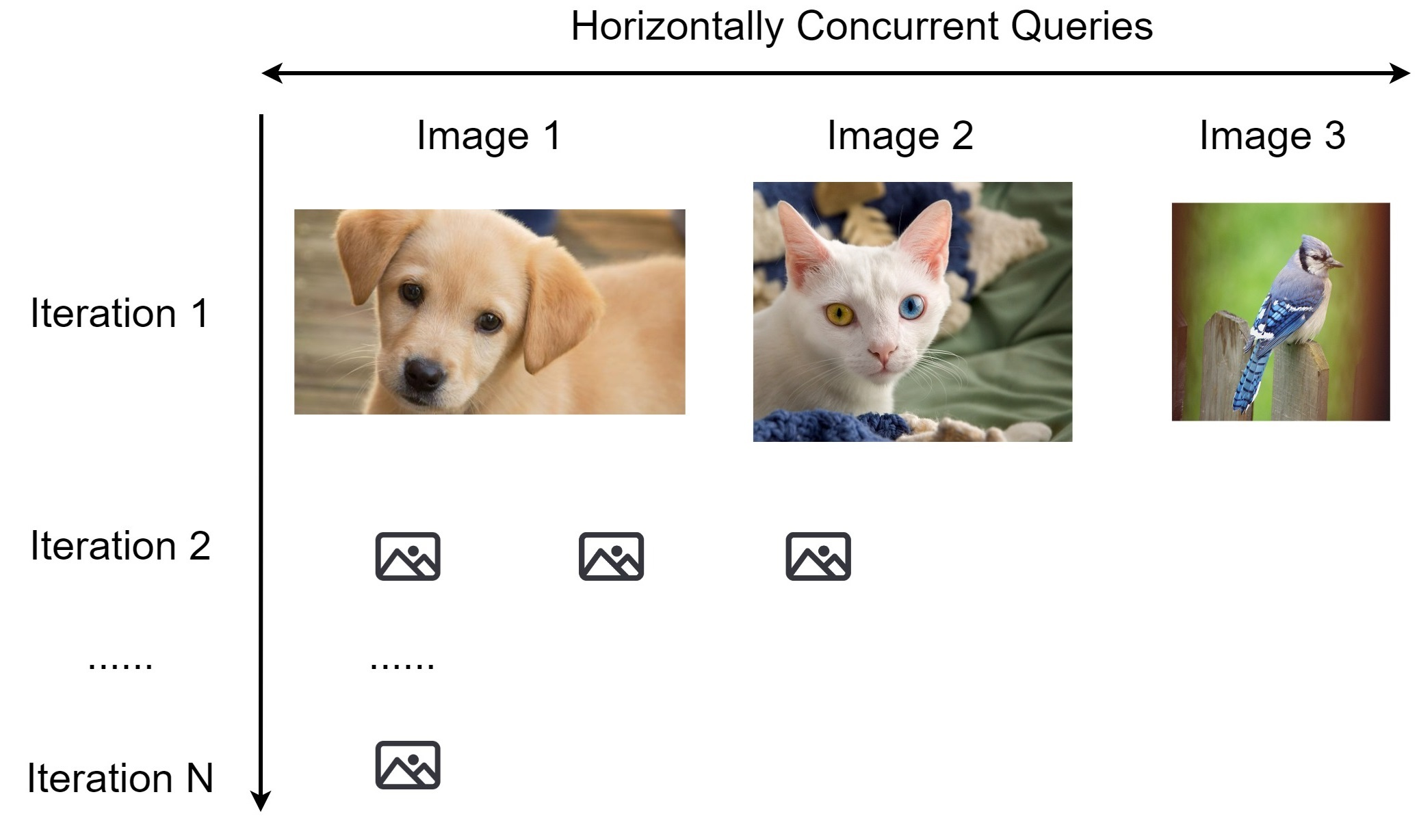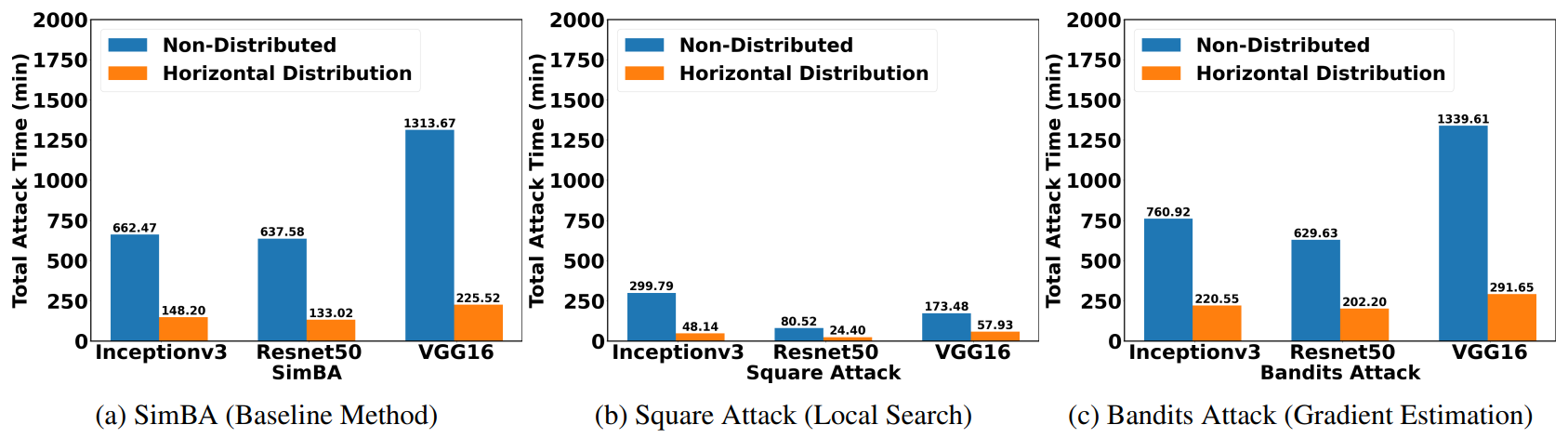Horizontal distribution reduces the total attack time by a factor of five.

### Vertical Distribution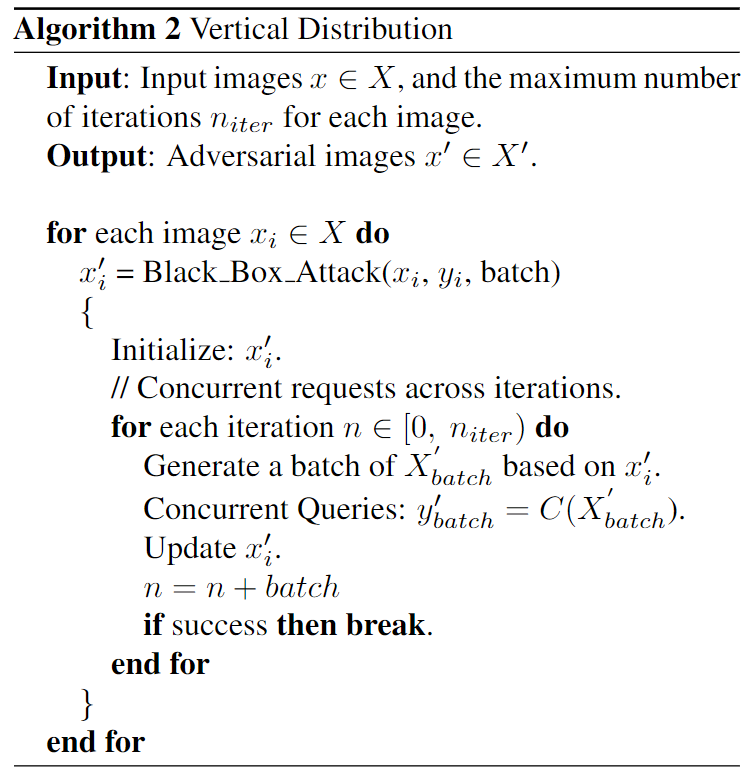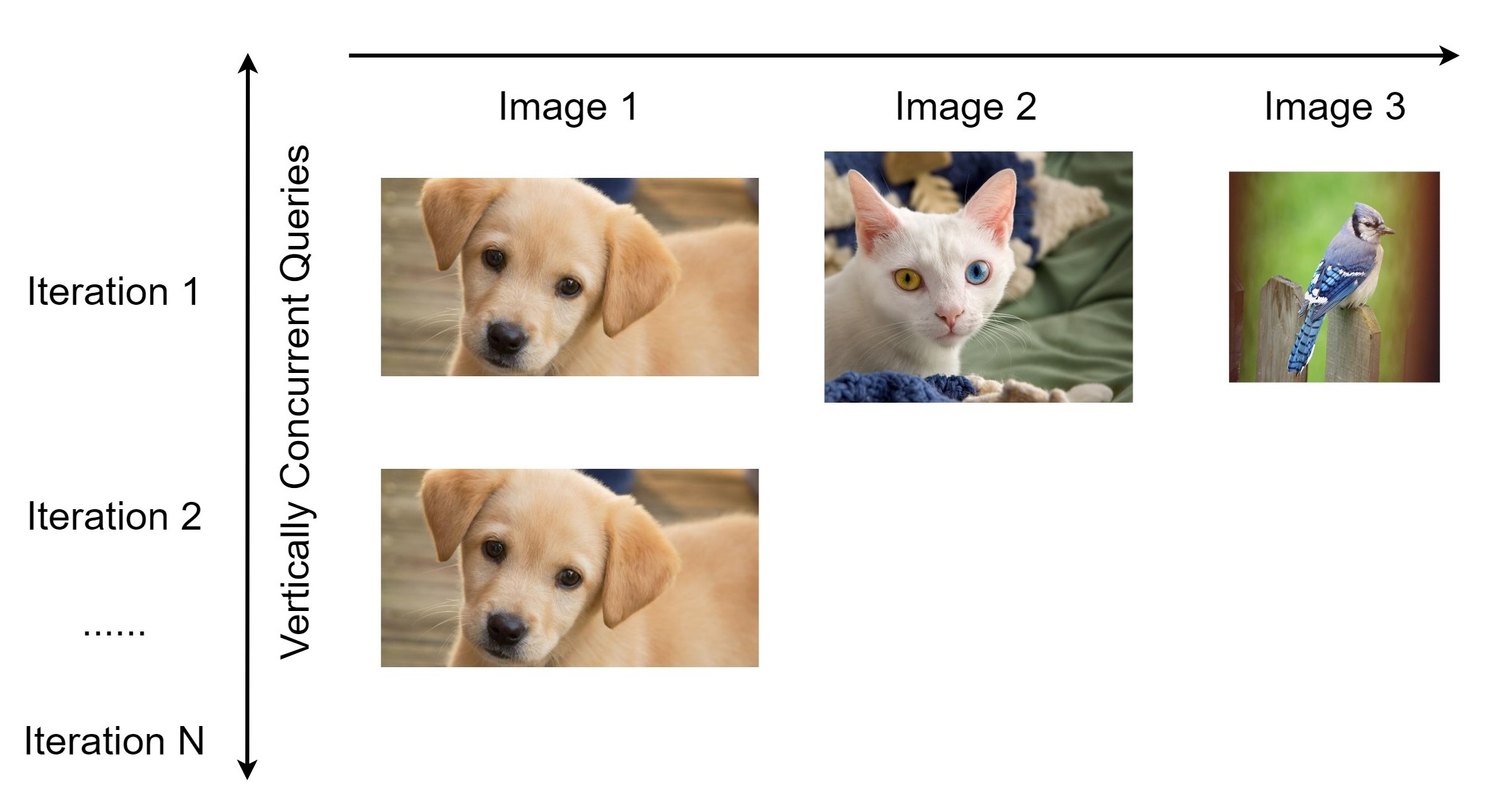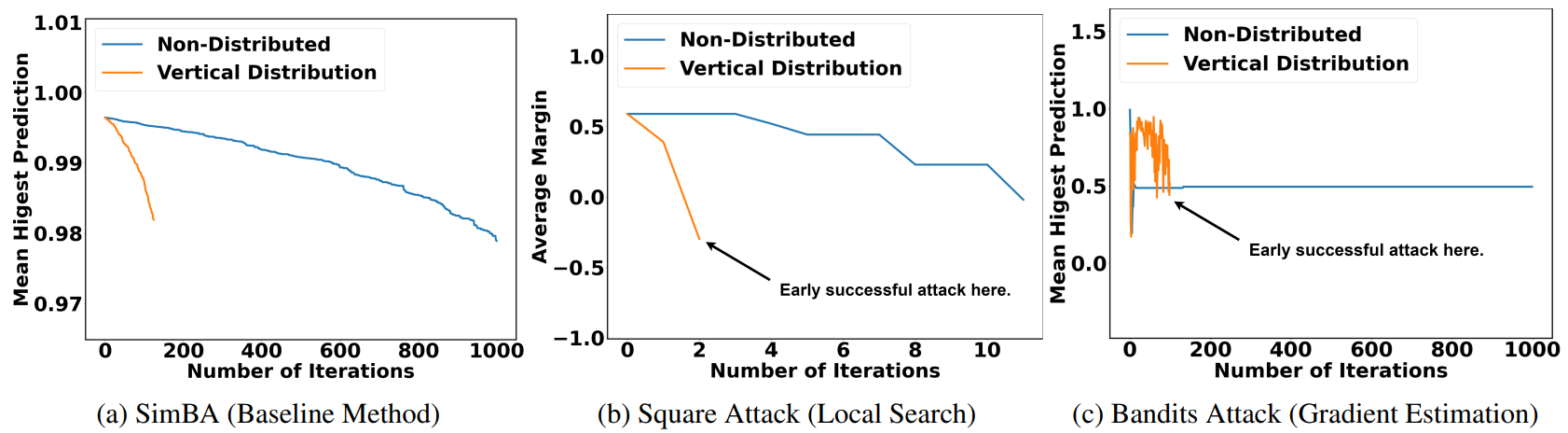Vertical distribution achieves succeesful attacks much earlier.

#### Conclusion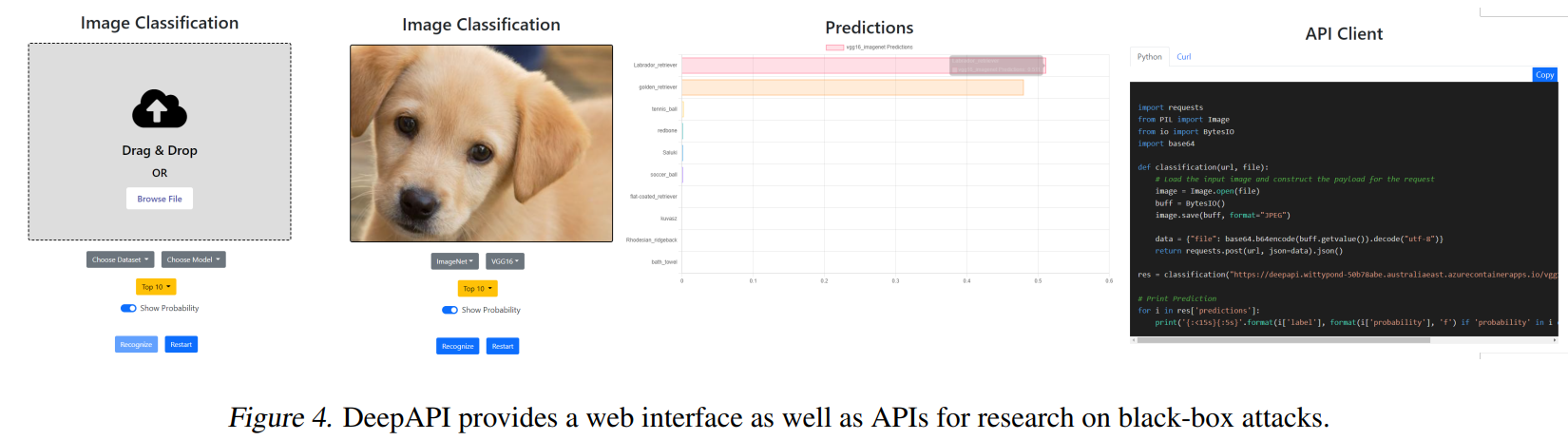### The Man-in-the-Middle Attack

A Hardware Attack against Object Detection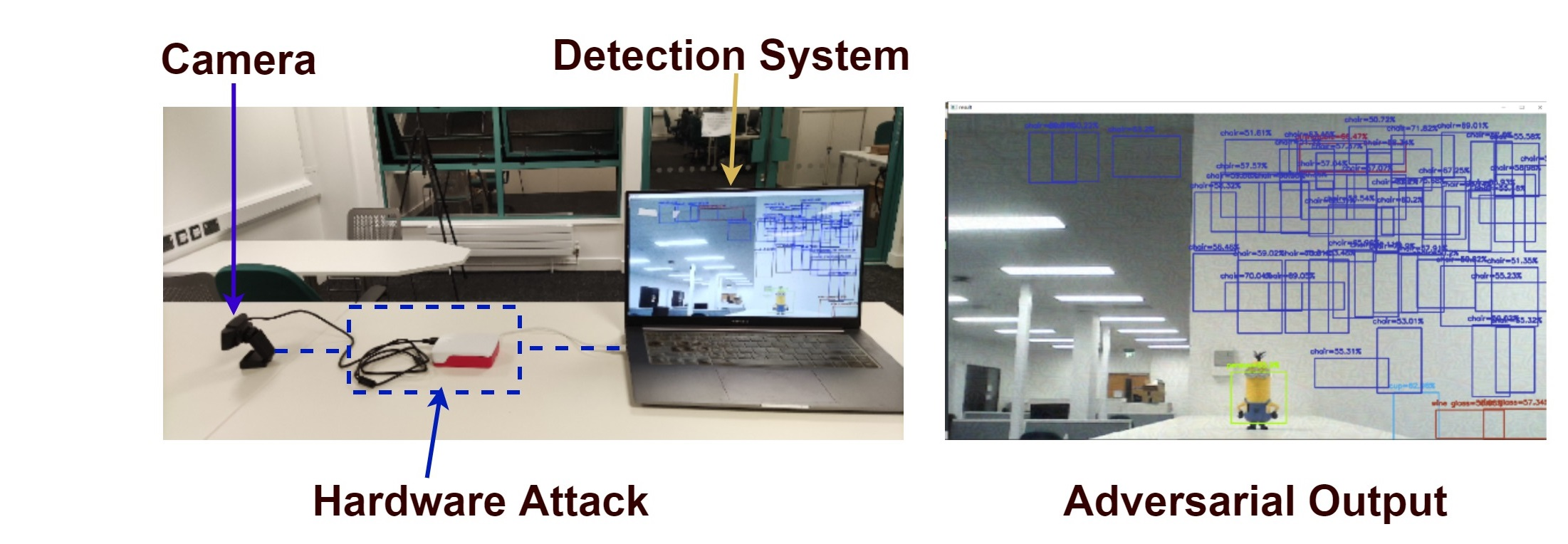Deep learning models are vulnerable to adversarial attacks.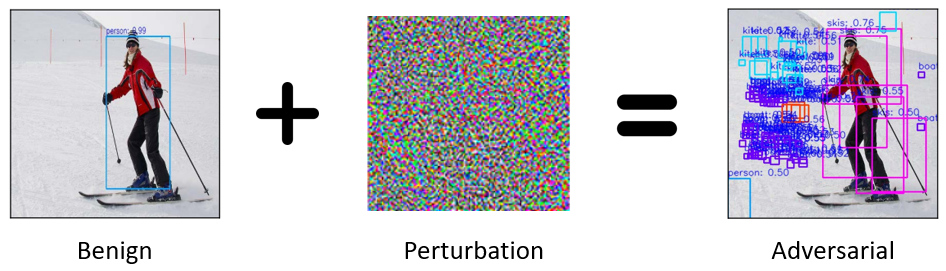To achieve real-time adversarial attacks, we need to solve two problems:

• How to generate the perturbation? (The PCB Attack)
• How to apply the perturbation? (The Man-in-the-Middle Attack)
###### Step 1: Generating the perturbation (The PCB Attack)

• Objective:
• $$\min_{\mathcal{W}} \ \mathcal{L}_{train} = f(\mathcal{W}; x, \mathcal{O}) \;\;\;\; \max_{x} \ \mathcal{L}_{adv} = f(x; \mathcal{O}^{\ast}, \mathcal{W})$$
• $$\mathcal{L}_{PC}(x) = \sum{\sigma(c_i) * \sigma(p_i)} \;\;\;\; \mathcal{L}_{PCB}(x) = \frac{\sum{(\sigma(c_i) * \sigma(p_i)}}{\sum{[\sigma(w_i) * \sigma(h_i)]^2}}$$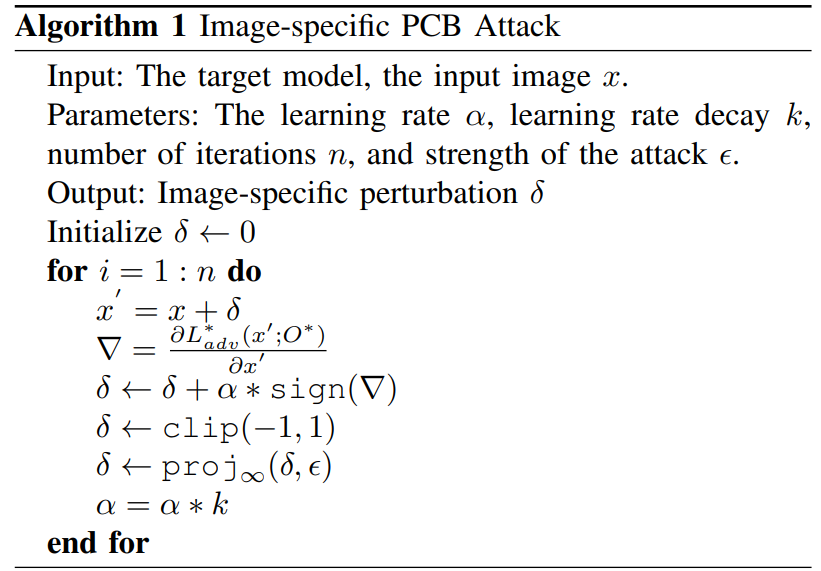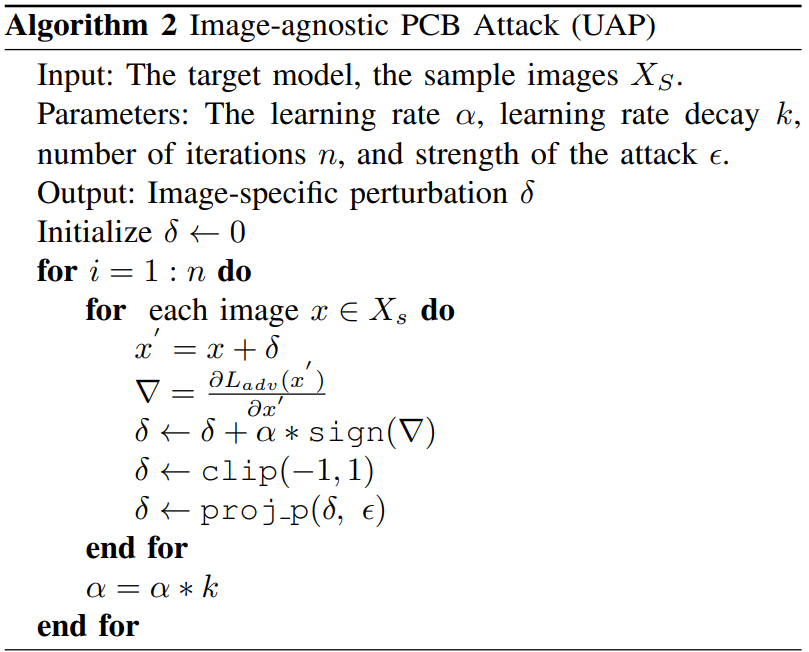###### Step 1: Generating the perturbation (The PCB Attack)

Prior Research                                                             Our Method

No learning rate decay                                                 With learning rate decay

Our method generates more bounding boxes, and have less variation.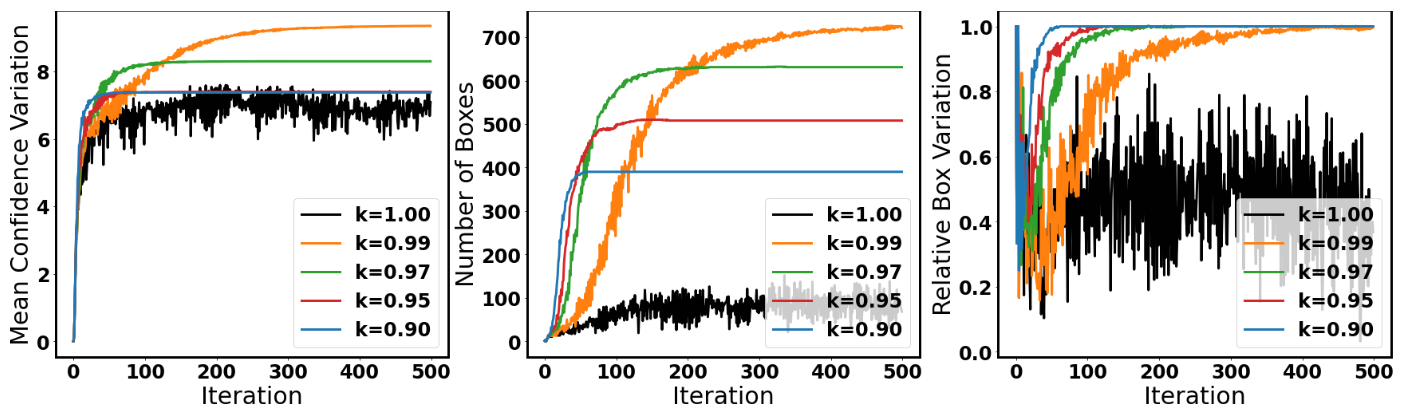###### Step 2: Applying the perturbation (The Man-in-the-Middle Attack)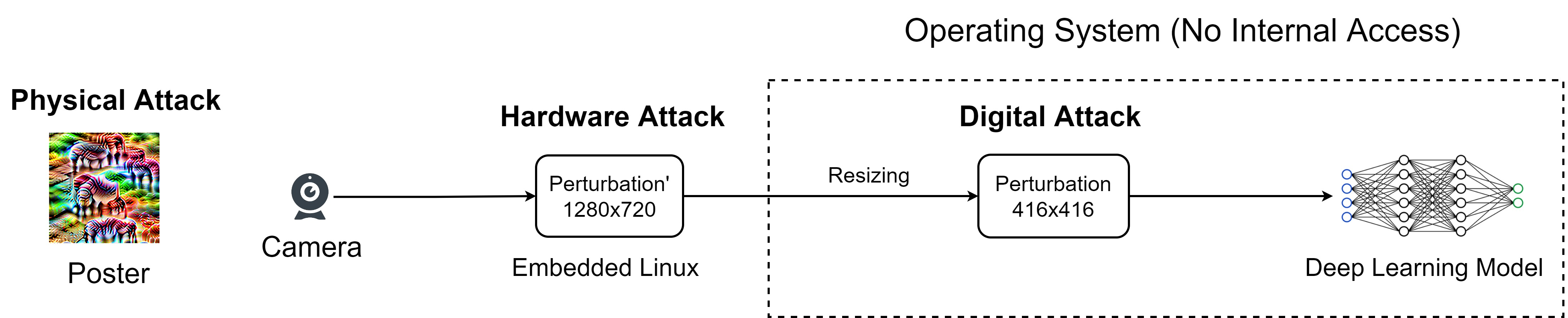### Is Deep Learning Secure for Robots?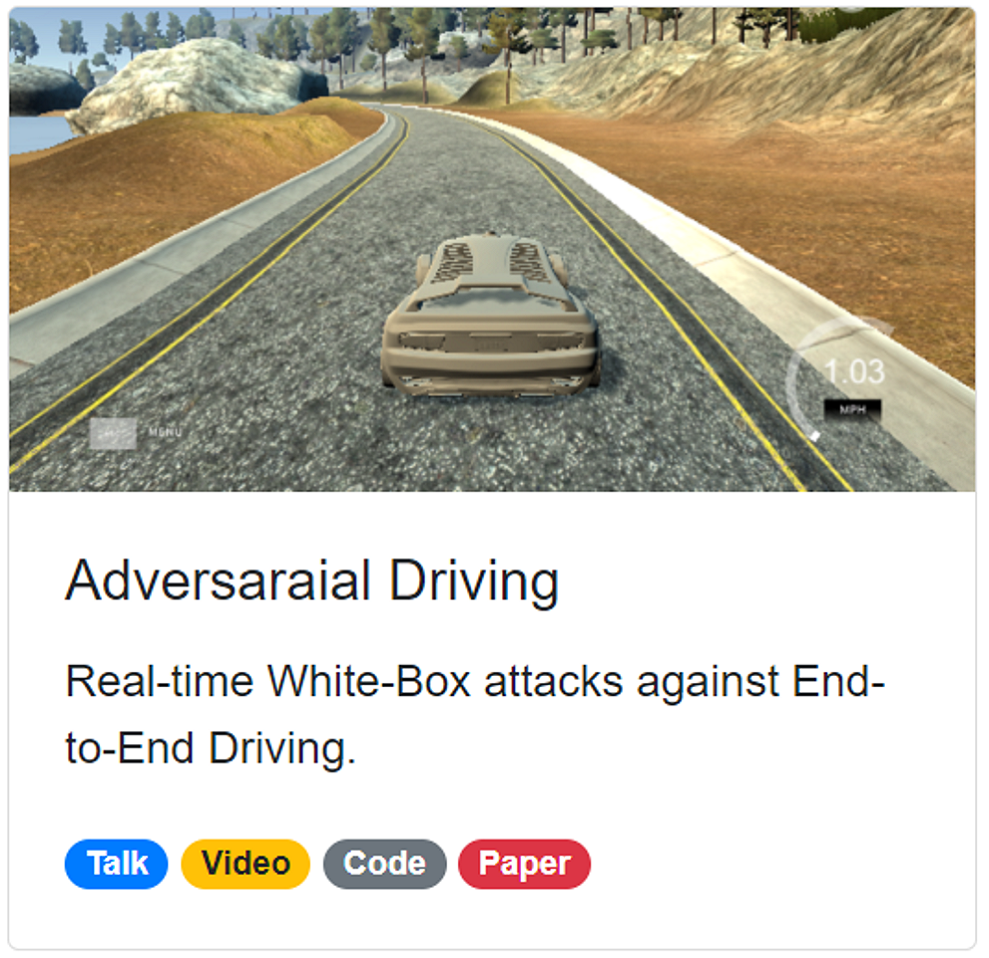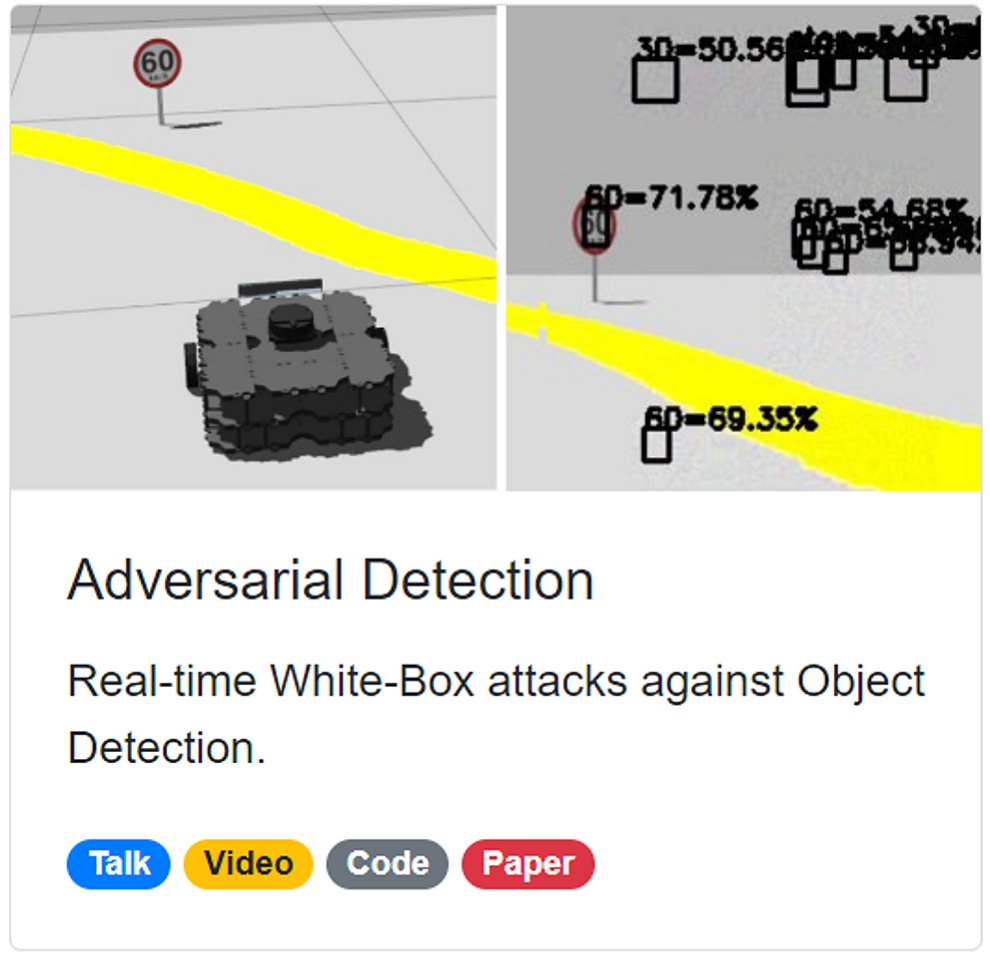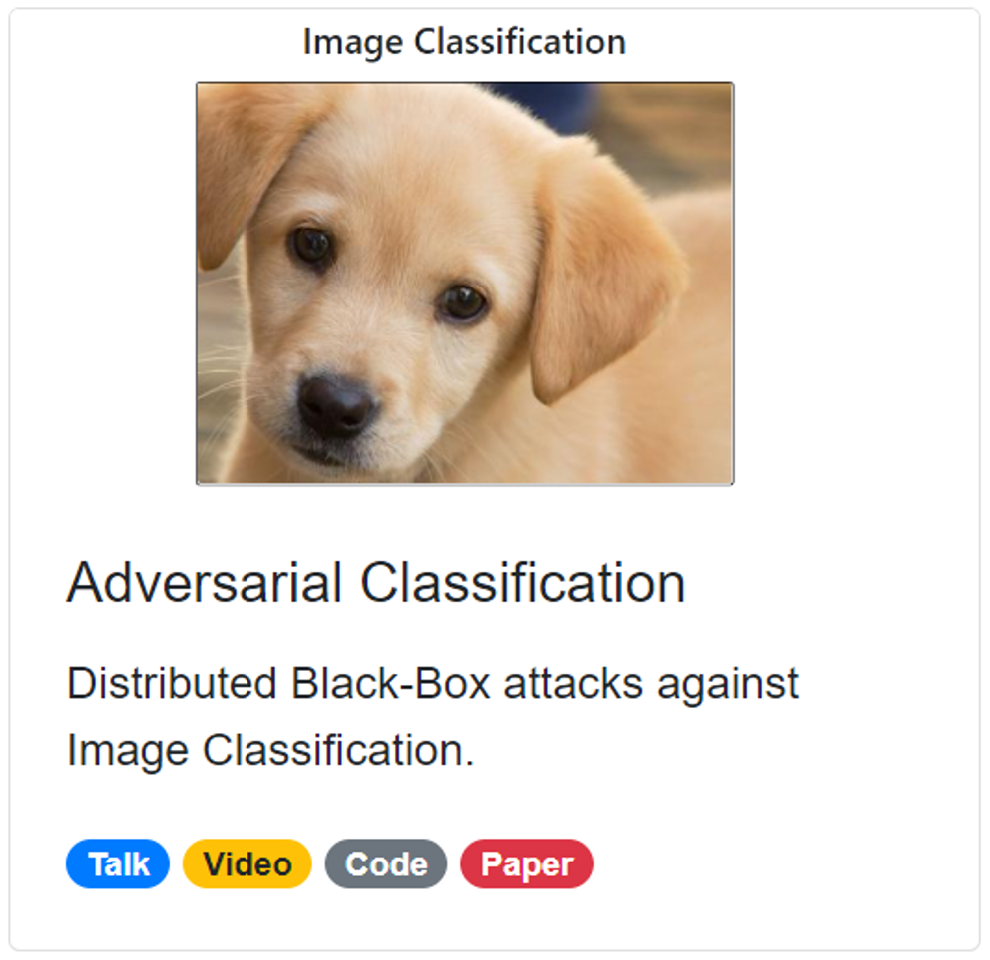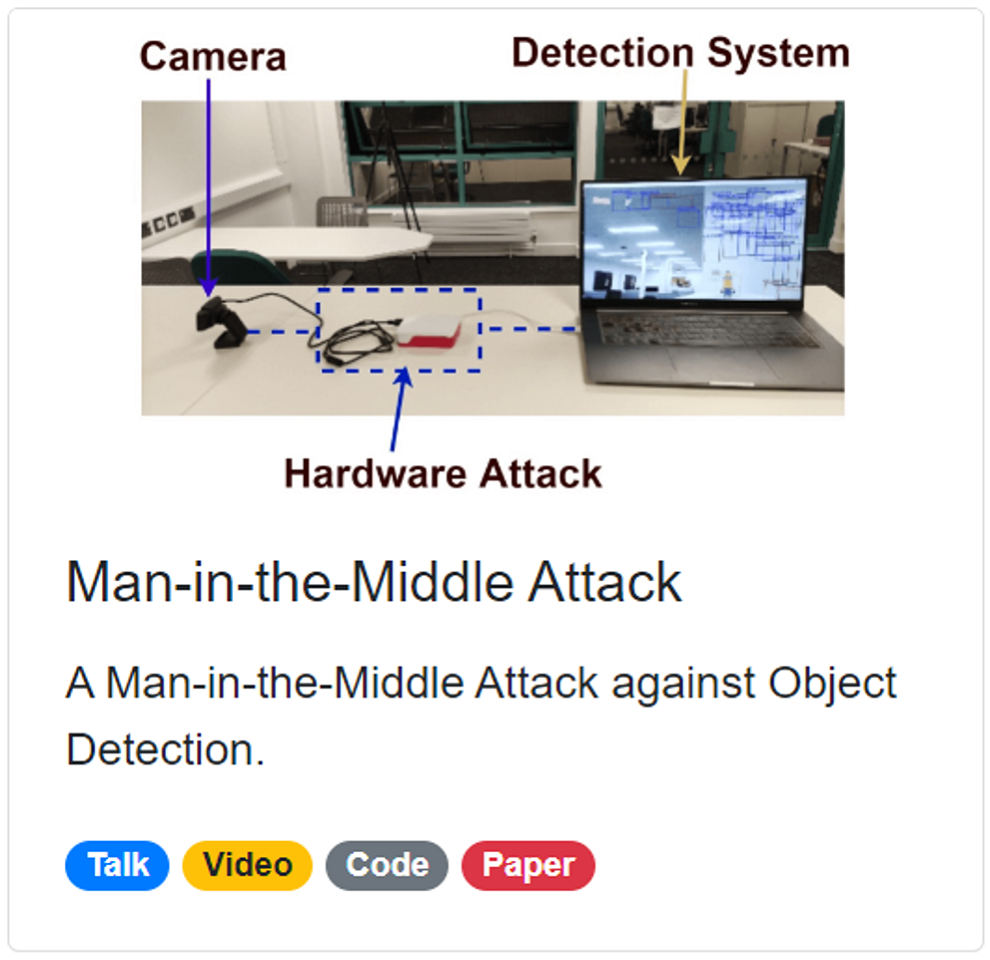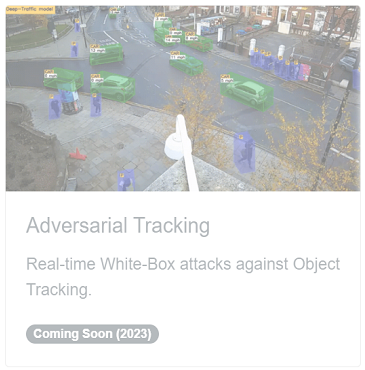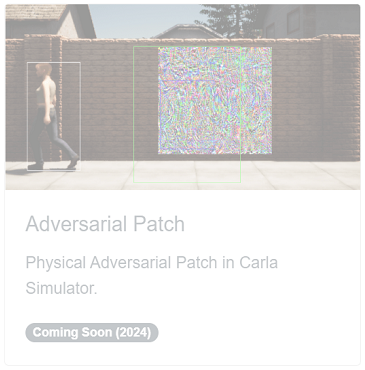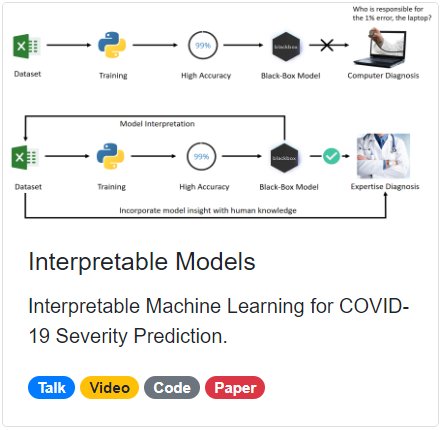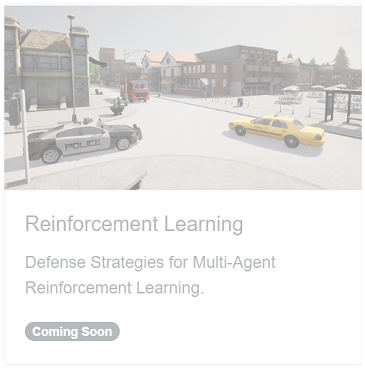## Thanks